@Duanxx 2016-07-31T09:01:56.000000Z 字数 36240 阅读 6507

# 支持向量机（SVM）

监督学习

@author : duanxxnj@163.com
@time : 2016-07-31

## 一、最大间隔分类器

### 1.2 最优决策面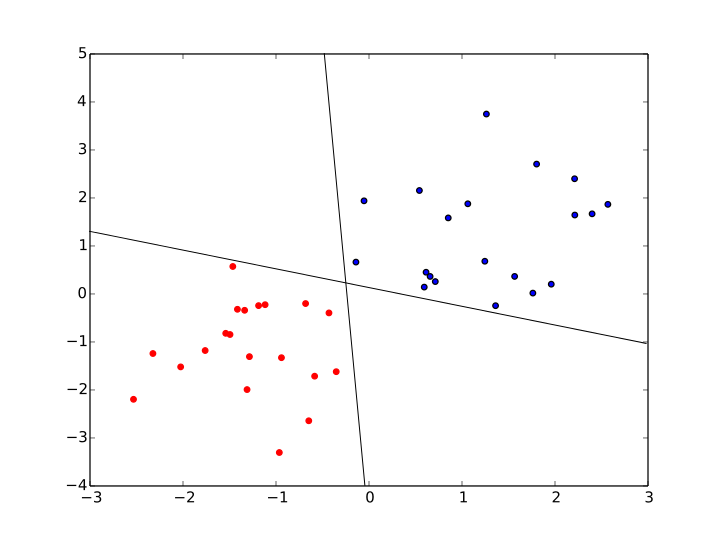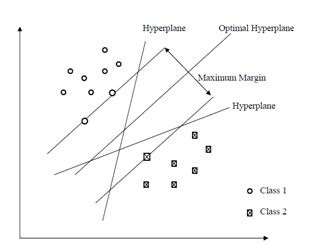### 1.3 最小间隔

$t_n=+1$的时候，如果样本被正确分类， $w^T\phi(x_n) + b>0$，上述样本点到决策面的间隔 $r_n$ 取正值
$t_n=-1$的时候，如果样本被正确分类， $w^T\phi(x_n) + b<0$，上述样本点到决策面的间隔 $r_n$ 仍然取正值

### 1.4 最小间隔最大化

$w$$b$比值，决定了一个决策面的点集，也就决定了一个决策面。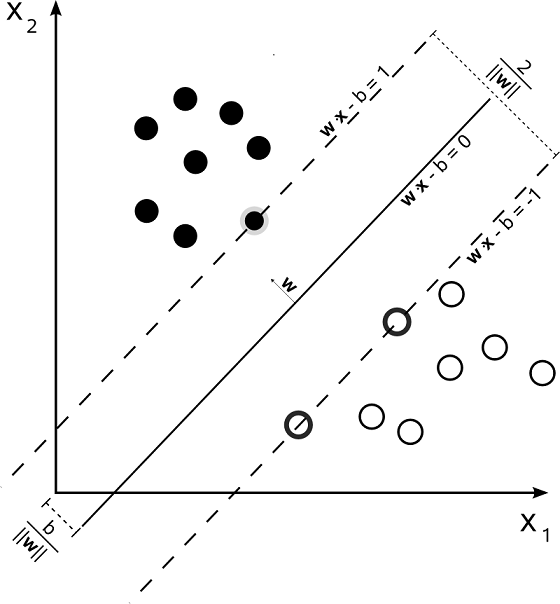### 1.5 拉格朗日对偶性

- 目标函数对原始问题是极大化，对偶问题则是极小化
- 原始问题目标函数中的系数，是对偶问题约束不等式中的右端常数，而原始问题约束不等式中的右端常数，则是对偶问题中目标函数的系数
- 原始问题和对偶问题的约束不等式的符号方向相反
- 原始问题约束不等式系数矩阵转置后，即为对偶问题的约束不等式的系数矩阵
- 原始问题的约束方程数对应于对偶问题的变量数，而原始问题的变量数对应于对偶问题的约束方程数
- 对偶问题的对偶问题是原始问题

#### 1、原始问题

• 如果不等式约束 $c_i(x) < 0$ ，等式约束 $h_j(x)=0$ ，那么上式最大化就会使得 $\alpha_i = 0$$\beta_j$ 可以取任意值。 此时所有满足约束条件的，并且最大化结果为$\theta_p(x)= f(x)$
• 如果不等式约束 $c_i(x) = 0$ ，等式约束 $h_j(x)=0$，那么上式最大化就会使得 $\alpha_i >0$ ,$\beta_j$ 可以取任意值。此时是满足约束条件的，并且最大化结果为$\theta_p(x)= f(x)$
• 如果存在不等式约束 $c_i(x) > 0$ , 等式约束 $h_j(x)=0$，即此时存在不等式约束违反约束条件，那么要使得上式最大化，必然会使得 $\alpha_i = \infty$ ，进而使得 $\theta_p(x)= \infty$
• 如果不等式约束 $c_i(x) \le 0$ , 存在 $h_j(x) \neq 0$ ，那么要使得上式最大化，必然会使得 $\beta_j = \infty$ ，进而使得 $\theta_p(x)= \infty$

### 1.6 最小间隔最大化求解

#### 求解内部极小化

$w=\sum_{i=1}^N{\alpha_it_i\phi(x_i)}$ 带入到 $L(w,b,\alpha)$ 中，就可以得到：

#### 求解外部极大化

• 在预测的时候，$w^*$$b^*$ 仅仅依赖于训练集合中 $\alpha_i^* > 0$ 的那些样本点，而其他样本点对$w^*$$b^*$ 没有影响。但是，为了求得 $w^*$$b^*$ ，在训练阶段，还是需要整个样本集合。也就是说，在做预测的时候，支持向量机需要的内存空间是非常小的，只需要存储支持向量即可，预测过程和非支持向量无关。
• 分类决策函数仅仅依赖于输入$x$ 和 训练样本之间的內积。这个內积是后面核函数的雏形，也是SVM得以广泛应用的关键。

### 1.7 SVM、LDA、Logistics Regression 算法比较

#### 对于Linear Discriminant Analysis

LDA假设$f_k(x)$是均值不同，方差相同的高斯分布，所以其类条件概率密度函数可以写为：

LDA和前面提到的Logistics Regression采用的单调变换函数一样，都是logit 函数：$log[p/(1-p)]$，对于二分类问题有：

## 二、软间隔最大化分类器

### 2.1 软间隔最大化分类器的两个问题

1.在SVM中，对于非线性可分的数据而言，哪些样本点是存在分类错误的样本点？
2.对于分类错误的点，软间隔最大化分类器 如何处理以得到相对比较好的决策面？

#### 哪些是分类错误的样本点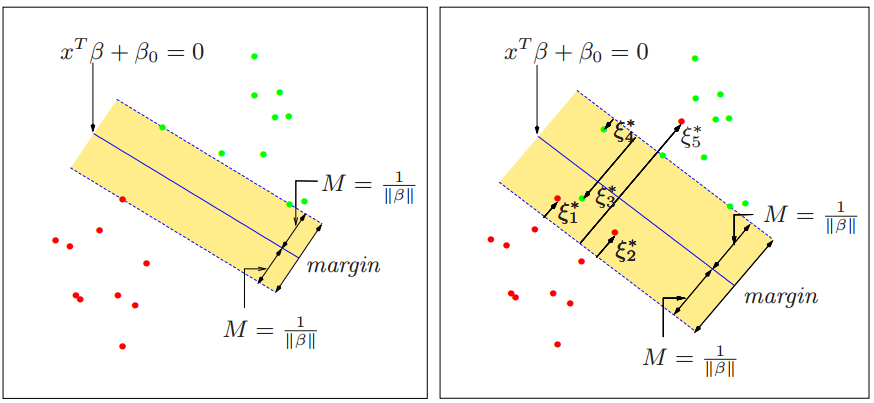1.非支持向量，即是在 $margin$ 两侧，被正确分类的样本点。这些样本点对于SVM的决策面没有贡献。
2.在$margin$边界上面的点，这个是支持向量，且被正确分类。
3.在$margin$内部，被正确分类的点，比如$\xi_1^*,\xi_2^*,\xi_4^*$，这些也是支持向量。
4.在决策面上的点，这些点可以拒绝判断，也可以直接认为是分类正确的点。
5.真正的被分类错误的点：$\xi_3^*,\xi_5^*$，这些点直接走到了决策面的另一边去了。

#### 分类错误的样本点的处理:软间隔变量

• 对于前面第三种情况：在$margin$内部，被正确分类的点，比如$\xi_1^*,\xi_2^*,\xi_4^*$。这些点的间隔为：

• 对于前面第四种情况：在决策面上的点，这些点的间隔为：

• 对于前面第五种情况：真正的被分类错误的点：$\xi_3^*,\xi_5^*$，这些直接走到了决策面的另一边去了的点，这些点的间隔为：

### 2.2 软间隔最大化分类器 的优化公式

• C 越小，模型越偏向于最小化$||w||^2$，而忽略$\sum_{i=1}^N \xi_i$，这样模型非常简单，但模型的错误率相对较高。
• C 越大，模型越偏向于最小化$\sum_{i=1}^N \xi_i$，而忽略$||w||^2$ ，这样模型的错误率相对较低，但模型相对较为复杂。

### 2.5 对参数 $\alpha,\xi$ 的讨论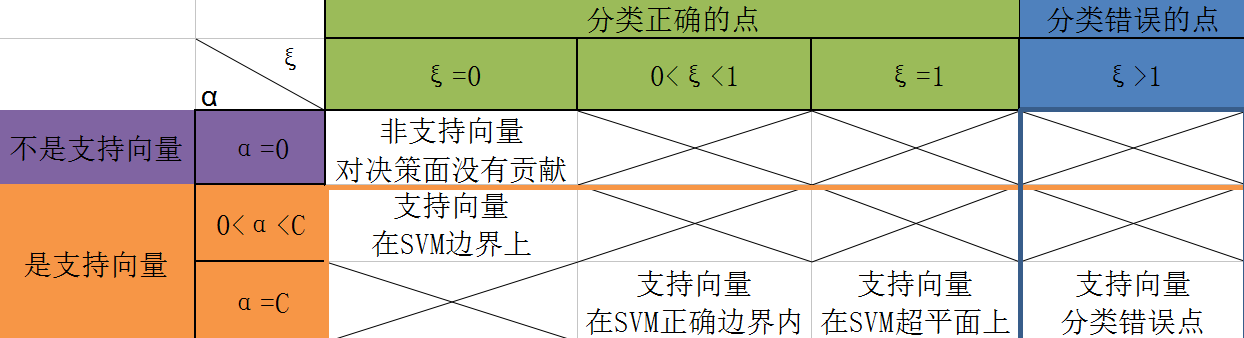- 当$\alpha_i =0$ 时，对应的样本点是非支持向量；当$\alpha_i >0$ 时，对应的样本点是支持向量
- 当$\xi \le1$ 时，对应的样本点可以被正确的分类；当$\xi >1$ 时，对应的样本点分类错误

1.当$\xi_i=0,\alpha_i=0$时，此时的样本点 $x_i$ 并不是支持向量，其被完全的分类正确，样本点满足：$t_i\{w^T\phi(x_i)+b\} > 1$

2.当$\xi_i=0,0<\alpha_i时,此时样本点刚好在SVM的间隔边界上，是支持向量，被正确分类，样本点满足：$t_i\{w^T\phi(x_i)+b\} = 1$

3.当$0<\xi_i<1,\alpha_i=C$时，此时样本点在SVM的正确的间隔边界内，是支持向量，被正确分类，样本点满足：$0< t_i\{w^T\phi(x_i)+b\} < 1$

4.当$\xi_i=1,\alpha_i=C$时，此时样本点在SVM决策面上，是支持向量，被正确分类，样本点满足：$t_i\{w^T\phi(x_i)+b\} = 0$

5.当$\xi_i>1,\alpha_i=C$时，是支持向量，被错误分类，样本点满足：$t_i\{w^T\phi(x_i)+b\} < 0$

• 在SVM间隔区间外的点，对应的$\alpha_i=0$
• 在SVM间隔区间边缘上的点，对应的$0<\alpha_i
• 在SVM间隔区间内部的点，对应的$\alpha_i=C$

## 三、基于核函数的非线性SVM

$\phi(x_i)$所代表的就是一种空间映射，是核技巧必备的一个元素，这也是为什么本文从一开始推导SVM的公式的时候，使用的就是$\phi(x)$而不是单纯的$x$的原因。

$<\phi(x_i),\phi(x)>$ 指的是 $\phi(x_i),\phi(x)$ 的內积。这里可以看到，SVM的判别函数最终变成了$\phi(x_i),\phi(x)$的內积的求解。

### 3.1 特征映射解决非线性可分问题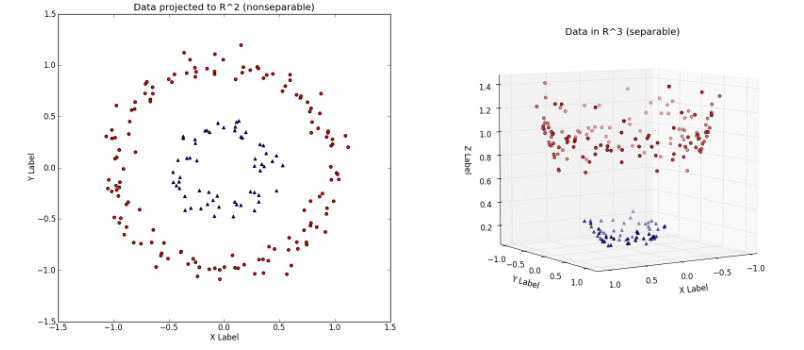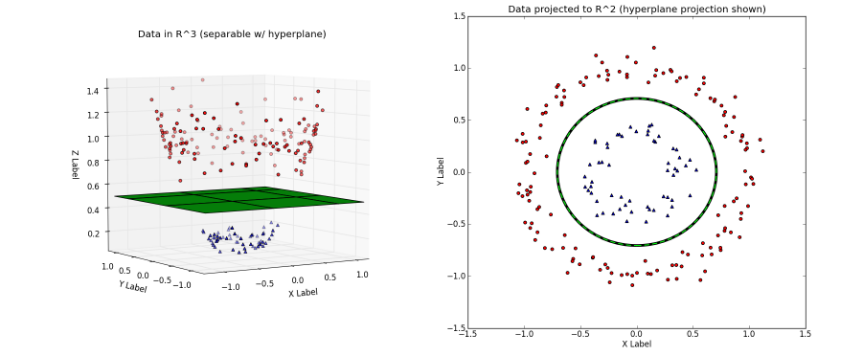### 3.3 非线性映射定$\phi(x)$与核函数$K(x_1,x_2)$的关系

• 对于给定的函数$K(x_1,x_2)$，其映射后的空间$Z$，可以使多样的，即可以映射到不同的特征空间中
• 对于给定的函数$K(x_1,x_2)$，即便映射到相同维度的特征空间，其映射函数$\phi(x)$也存在多样性

1. 取特征空间$Z=R^3$，即维度升高一个维度，此时$\phi(x)$可以取：

1. 取特征空间$Z=R^4$，即维度升高两个维度，此时$\phi(x)$可以取：

### 3.4 常用的核函数

1.Polynomial 核函数：

2.Gaussian 核函数：

3.Sigmoid 核函数：

4.RBF 核函数：